Introduction to eigenvalues and eigenvectors:

As we saw in the simple succession model, after a long enough time period, the fraction of the landscape that each vegetation type occupies approaches some constant. In the simplest model, all the land moved to the "climax" state - so the vector that described the landscape was [0 0 1] for the case of 3 landscape types where the last type is the climax state. However, when we added disturbance (fire) to the system, the state of the system again approached some fixed fraction of each vegetation type, but all types were present. What we want to do now is define a mathematical method to find these "final" states - the jargon for this is that we are finding an "eigenvector" for the system. This

means a vector that characterizes the system after a long time period has elapsed. It is a vector the elements of which tell us what fraction of the landscape each vegetation type will have after a long time. This is also called the long-term equilibrium state of the vegetation.

One way to find an eigenvector is numerically. We can use Matlab to find for any transition matrix P, Pn , where n is a large number (say 100), then multiply this times the initial vector for the landscape, x0, to get a numerical answer for the long-term state of the landscape. If the initial vector x0 contained the fraction of the landscape in each vegetation type, then P100 x0 will be a vector giving the long-term fraction of the landscape in each vegetation type. If the initial vector x0 rather represents the number of hectares or acres of each type, then divide each term in P100 x0 by the sum of the components of this vector to get the long-term fraction of the landscape in each state (the eigenvector is specified only up to a constant multiple).

A second way to get the eigenvector is to realize that it arises when the long-term structure of the system doesn't change. This is expressed as P y = l y where l is a constant that represents how the vector of vegetation types increases or decreases through one time period. In our case of a fixed landscape, land area is neither created nor destroyed, so there is no change from one time period to another and so l = 1. This means that to find the eigenvector all we need to do is find a vector y that satisfies P y = y. This is easy to do using simple algebra for small matrices, but for larger ones the mathematics becomes more difficult. In this case you either use the theory of determinants, or else use Matlab to find the answer.

Consider the 2x2 matrix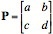and lets look at the equations arising from P y = l y where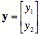This holds if a y1 + b y2 = l y1  and c y1 + d y2 = l y2. In order for these to both hold, we need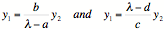So the only way these can both hold is if y1=y2=0 (which is not interesting) or if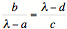This is a quadratic equation in lambda: l2 - (a+d)l + ad - bc = 0 and this equation is called the characteristic equation for this matrix. We call a+d the Trace of the matrix P ( Tr(P) ) and ad-bc the determinant of the matrix P

( Det(P) ). By solving this quadratic for the roots, we find the eigenvalues l (there will be two in general for a 2x2 matrix). To find the eigenvector, we plug in one of these l values to find the ratio of y1 to y2, and this gives us the eigenvector (it is only known up to a constant multiple).

Note that in the case of our succession model where the landscape area doesn't change, we have l = 1, and so we must have a+d = ad-bc. You should check for yourselves that this holds true because the columns of the matrix P sum to one (so a+d=1 and b+c=1). So then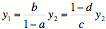gives the ratio of the elements of the eigenvector. Then the long-term fraction of the landscape in each of the two vegetation classes is obtained by normalizing the vector y so it’s components sum to 1.